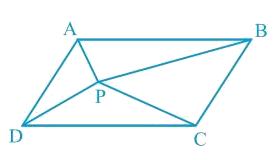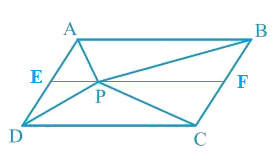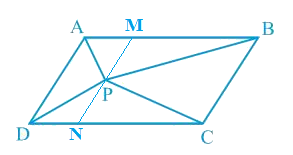# Ex.9.2 Q4 Areas of Parallelograms and Triangles Solution - NCERT Maths Class 9

Go back to  'Ex.9.2'

## Question

In the given figure, $$P$$ is a point in the interior of a parallelogram $$ABCD$$. Show that

(i)

\begin{align}ar (APB)\!+\!ar (PCD)\!=\!\frac{1}{2} ar(ABCD)\end{align}

(ii)

$$ar(APD)\!+\!ar(PBC)\!=\!ar(APB)\!+\!ar(PCD)$$

[Hint: Through. $$P$$, draw a line parallel to $$AB$$]Video Solution
Areas Of Parallelograms And Triangles
Ex 9.2 | Question 4

## Text Solution

What is known?

$$P$$ is a point in the interior of a parallelogram $$ABCD.$$

What is unknown?

How we can show that

(i)$$ar(APB)\!+\!ar(PCD)\!=\!\frac{1}{2}ar(ABCD)$$

(ii) $$ar (APD)\!+\!ar(PBC)\!=ar(APB)\!+\!ar(PCD)$$

Reasoning:

Draw a line parallel to and $$CD$$ passes through point $$P$$. Now we can observe the figure, if a triangle and parallelogram are on same base and between same parallel lines then area of triangle will be half of area of parallelogram so we can find area of triangles $$APB$$ and $$PCD$$ to get the required result. Similarly, we can draw a line parallel to $$AD$$ and $$BC$$ to get the required result.

Steps:(i) Let us draw a line segment $$EF$$, passing through the point $$P$$ and parallel to line segment $$AB$$ in parallelogram $$ABCD$$,

$$AB\; || \;EF$$ (By construction) ... (1)

We know that, $$ABCD$$ is a parallelogram.

$$\therefore AD\; ||\; BC$$ (Opposite sides of a parallelogram are parallel)

$$AE\; || \;BF$$ ... (2)

From Equations (1) and (2), we obtain

$$AB\; || \;EF$$ and $$AE\; || \;BF$$

Therefore, quadrilateral $$ABFE$$ is a parallelogram. Similarly , it can be deduced that quadrilateral $$EFCD$$ is a parallelogram.

It can be observed that $$\Delta APB$$ and parallelogram $$ABFE$$ are lying on the same base $$AB$$ and between the same set of parallel lines $$AB$$ and $$EF$$.

\begin{align}\therefore \!\text{Area}(\Delta {APB})\!=\!\frac{1}{2} \text{Area}({ABFE})\dots(3)\end{align}

Similarly, for $$\Delta PCD$$ and parallelogram $$EFCD$$,

\begin{align}\therefore \rm{Area}(\Delta{PCD})\!=\!\frac{1}{2}\text{Area} (EFCD) \dots(4)\end{align}

Adding Equations ($$3$$) and ($$4$$), we obtain

\begin{align}\begin{bmatrix}\text{Area }(\Delta {APB})+\\ \text { Area }(\Delta{PCD})\end{bmatrix}=\frac{1}{2} \begin{bmatrix}\text { Area }({ABFE})+\\\text { Area }({EFCD})]\end{bmatrix}\end{align}

\begin{align}\begin{bmatrix}\text{Area }\left(\Delta {APB}\right)+\\\text { Area }(\Delta {PCD})\end{bmatrix}=\frac{1}{2}\text { Area }({ABCD})\rm{ ...(5)}\end{align}

(ii)Let us draw a line segment $$MN$$, passing through point $$P$$ and parallel to line segment $$AD$$. In parallelogram $$ABCD$$,

$$MN \;|| \;AD$$ (By construction) ... (6)

We know that, $$ABCD$$ is a parallelogram.

$$\therefore$$  $$AB\; ||\; DC$$ (Opposite sides of a parallelogram are parallel)

$$AM\; ||\; DN$$ … (7)

From Equations (6) and (7), we obtain

$$MN \;|| \;AD$$ and $$AM\; ||\; DN$$

Therefore, quadrilateral $$AMND$$ is a parallelogram.

It can be observed that $$\Delta APD$$ and parallelogram  are lying on the same base  and between the same parallel lines  and .

\begin{align}\therefore \text{Area }\!(\Delta {APD})\!=\!\frac{1}{2} \text {Area} (AMND) \ldots(8)\end{align}

Similarly, for $$\Delta PCB$$ and parallelogram ,

\begin{align}\therefore \rm{Area}\,(\Delta {PCB})\!=\!\frac{1}{2}\text { Area }({MNCB})...(9)\end{align}

Adding Equations () and (), we obtain

\begin{align}\begin{bmatrix}\text{Area }\left(\Delta {APD}\right)+\\\text { Area }(\Delta {PCB})\end{bmatrix}= \frac{1}{2}\begin{bmatrix} \text { Area }({AMND})+\\\text { Area }({MNCB})\end{bmatrix}\end{align}

\begin{align}\begin{bmatrix}\text{Area }\left(\Delta {APD}\right)+\\\text { Area }(\Delta {PCB})\end{bmatrix}=\frac{1}{2} \text { Area }({ABCD})...(10) \end{align}

On comparing Equations () and (), we obtain

\begin{align}\begin{bmatrix}\rm{Area}\,\,(\Delta {APD})+\\\text { Area }(\Delta {PBC})\end{bmatrix}=\begin{bmatrix}\text { Area }(\Delta {APB})+\\\text { Area }(\Delta {PCD})\end{bmatrix}\end{align}

Learn from the best math teachers and top your exams

• Live one on one classroom and doubt clearing
• Practice worksheets in and after class for conceptual clarity
• Personalized curriculum to keep up with school